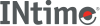﻿ cos, coshINtime SDK Help
cos, cosh

Cos calculates the cosine and cosh calculates the hyperbolic cosine of an angle.

```#include <math.h>

double cos (double x);

double cosh (double x);
```

`x`

#### Return Values

The cosine or hyperbolic cosine.

 cos Returns a PLOSS error if x is large and a partial loss of significance in the result occurs; function sets errno to ERANGE. Prints a TLOSS message to stderr and returns 0 if x is so large that significance in the result is completely lost; function sets errno to ERANGE. cosh Returns HUGE_VAL and sets errno to ERANGE if the result is too large.
Note:   This function does not return standard ANSI domain or range errors.

#### Requirements

Versions Defined in Include Link to
INtime 3.0 intime/rt/include/math.h math.h clib.lib